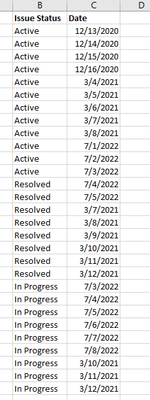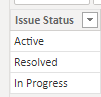cancel
Showing results for
Did you mean:Frequent Visitor

## I am not sure how to create a logic for this

A bit new to PBI. I want to create to Montly performance tracker ( # of issue active, closed, resolved per month), result will be as I shown in result table. And Data is looking like something I have in data table. Thanks in advance!

*Data Table**Result Table*

 Issue Status Current month Past 1 month Past X month # of Active issues 1 4 5 #Resovled Issues 2 3 4

1 ACCEPTED SOLUTIONCommunity Support

Hi @Crazydog ,

You can try this method:

Sample data is your data table and because of the data's date, I assume that the current data is July,2022.

First, calculate the month and the year of the date:New Column:

``Month = MONTH('Sample Table'[Date])``
``Year = YEAR('Sample Table'[Date]) ``

Then create a new table:

Issue Table:

``Issue Table = SUMMARIZE('Sample Table','Sample Table'[Issue Status])``Then new columns:

``````Current Month =
CALCULATE (
COUNT ( 'Sample Table'[Issue Status] ),
FILTER (
'Sample Table',
'Issue Table'[Issue Status] = 'Sample Table'[Issue Status]
&& 'Sample Table'[Month] = 7
&& 'Sample Table'[Year] = 2022
)
)
``````

In this sample, month is 7 and year is 2022, you can change them to the data you need, use the function like Today().

``````Past 1 Month =
CALCULATE (
COUNT ( 'Sample Table'[Issue Status] ),
FILTER (
'Sample Table',
'Issue Table'[Issue Status] = 'Sample Table'[Issue Status]
&& 'Sample Table'[Month] = 7 - 1
&& 'Sample Table'[Year] = 2022
)
)
``````
``````Past X Month =
CALCULATE (
COUNT ( 'Sample Table'[Issue Status] ) - SUM ( 'Issue Table'[Current Month] )
- SUM ( 'Issue Table'[Past 1 Month] ),
FILTER (
'Sample Table',
'Issue Table'[Issue Status] = 'Sample Table'[Issue Status]
)
)
``````

The result is:The Past 1 Month is blank because the sample data don't have the June data.

If I change the data in the code like this:

``````Past 1 Month =
CALCULATE (
COUNT ( 'Sample Table'[Issue Status] ),
FILTER (
'Sample Table',
'Issue Table'[Issue Status] = 'Sample Table'[Issue Status]
&& 'Sample Table'[Month] = 4 - 1
&& 'Sample Table'[Year] = 2021
)
)
``````There is the result of this column.

Is this what you expect?

Hope this helps you.

Best Regards,

Community Support Team _Yinliw

If this post helps, then please consider Accept it as the solution to help the other members find it more quickly.

4 REPLIES 4Frequent Visitor

What am I doing wrong here ?

Current month =
CALCULATE (
COUNT (v_issues_all_months_workorders[issue status]),
FILTER (
'v_issues_all_months_workorders',
'Issue_Table_1'[issue status] = v_issues_all_months_workorders[issue status]
&& v_issues_all_months_workorders[Month] = MONTH(TODAY()-1)
&& v_issues_all_months_workorders[Year] = YEAR(TODAY())
)
)

I am looking for the month - 1 from today and hence I did "MONTH(TODAY()-1)" but I am not getting correct result. Any help is appreciated!Community Support

Hi @Crazydog ,

You can try this method:

Sample data is your data table and because of the data's date, I assume that the current data is July,2022.

First, calculate the month and the year of the date:New Column:

``Month = MONTH('Sample Table'[Date])``
``Year = YEAR('Sample Table'[Date]) ``

Then create a new table:

Issue Table:

``Issue Table = SUMMARIZE('Sample Table','Sample Table'[Issue Status])``Then new columns:

``````Current Month =
CALCULATE (
COUNT ( 'Sample Table'[Issue Status] ),
FILTER (
'Sample Table',
'Issue Table'[Issue Status] = 'Sample Table'[Issue Status]
&& 'Sample Table'[Month] = 7
&& 'Sample Table'[Year] = 2022
)
)
``````

In this sample, month is 7 and year is 2022, you can change them to the data you need, use the function like Today().

``````Past 1 Month =
CALCULATE (
COUNT ( 'Sample Table'[Issue Status] ),
FILTER (
'Sample Table',
'Issue Table'[Issue Status] = 'Sample Table'[Issue Status]
&& 'Sample Table'[Month] = 7 - 1
&& 'Sample Table'[Year] = 2022
)
)
``````
``````Past X Month =
CALCULATE (
COUNT ( 'Sample Table'[Issue Status] ) - SUM ( 'Issue Table'[Current Month] )
- SUM ( 'Issue Table'[Past 1 Month] ),
FILTER (
'Sample Table',
'Issue Table'[Issue Status] = 'Sample Table'[Issue Status]
)
)
``````

The result is:The Past 1 Month is blank because the sample data don't have the June data.

If I change the data in the code like this:

``````Past 1 Month =
CALCULATE (
COUNT ( 'Sample Table'[Issue Status] ),
FILTER (
'Sample Table',
'Issue Table'[Issue Status] = 'Sample Table'[Issue Status]
&& 'Sample Table'[Month] = 4 - 1
&& 'Sample Table'[Year] = 2021
)
)
``````There is the result of this column.

Is this what you expect?

Hope this helps you.

Best Regards,

Community Support Team _Yinliw

If this post helps, then please consider Accept it as the solution to help the other members find it more quickly.Frequent Visitor

This is great! I appereciate your help, efforts and time you taken!Super User